### IMO Shortlist 1971 problem 8

Kvaliteta:
Avg: 0,0
Težina:
Avg: 0,0
Determine whether there exist distinct real numbers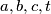$a, b, c, t$ for which:

(i) the equation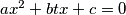$ax^2 + btx + c = 0$ has two distinct real roots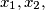$x_1, x_2,$

(ii) the equation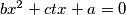$bx^2 + ctx + a = 0$ has two distinct real roots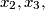$x_2, x_3,$

(iii) the equation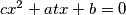$cx^2 + atx + b = 0$ has two distinct real roots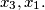$x_3, x_1.$
Izvor: Međunarodna matematička olimpijada, shortlist 1971